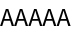Function Repository Resource:

VigenereDecipher

Decipher a Vigenère-enciphered string

Contributed by: Sander Huisman
 ResourceFunction["VigenereDecipher"][string,key] deciphers string using the key key. ResourceFunction["VigenereDecipher"][string,{key1,key2,…}] repeatedly deciphers string using first key1, then key2, etc. ResourceFunction["VigenereDecipher"][{s1,s2,…},key] deciphers the strings s1,s2, etc. using the key key. ResourceFunction["VigenereDecipher"][key] represents an operator form of ResourceFunction["VigenereDecipher"] that can be applied to an expression.

Details and Options

The key can be in either lowercase or uppercase letters.
Spaces in the key will leave letters unchanged, as do the letters A and a in the key.
Letters outside az and AZ are not transformed.
The case of the enciphered message is retained in the deciphered message.
When multiple keys are given and the LCM of the lengths of the keys is small, the keys get combined into a single key, which is then used to decipher string. This will, especially for long strings, yield faster results and give the same result as Fold[ResourceFunction["VigenereDecipher"],string,{key1,key2,}].
ResourceFunction["VigenereDecipher"][key][string] is equivalent to ResourceFunction["VigenereDecipher"][string,key].

Examples

Basic Examples

Decipher a message using the key "LEMON":

 In:=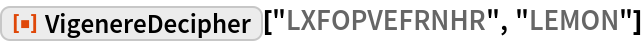Out=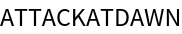Decipher with multiple keys:

 In:=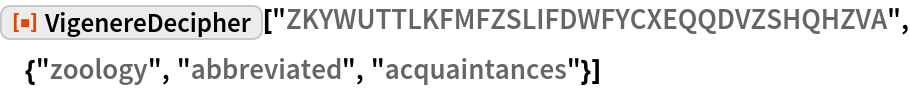Out=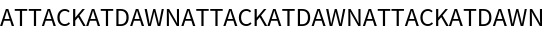Scope

Decipher a message twice, first using "GO" and then "CAT":

 In:=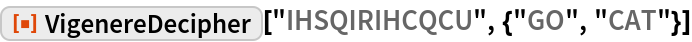Out=Decipher multiple strings:

 In:=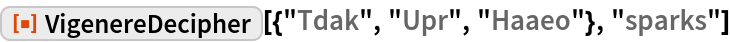Out=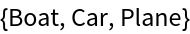Create an operator that deciphers strings with the key "sparks":

 In:=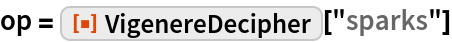Out=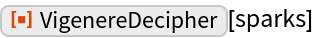Apply the operator to a string:

 In:=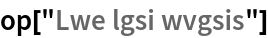Out=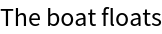Properties and Relations

If a single character is used, the Vigenère cipher is identical to a Caesar cipher:

 In:=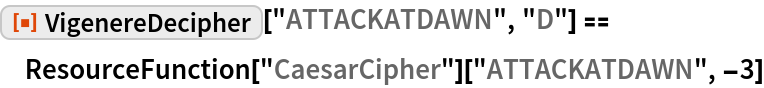Out=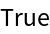When deciphering with multiple keys, the keys can first be enciphered with each other to decipher the message with a single key. The length of the replacing key has to be the LCM of the lengths of each of the keys:

 In:=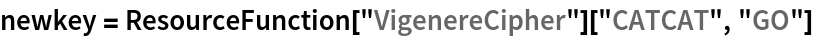Out=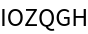This indeed matches with what you expect:

 In:=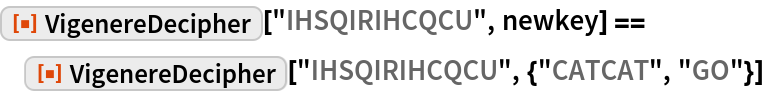Out=The case of the original text is retained; the case of the key does not matter:

 In:=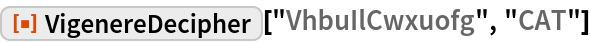Out=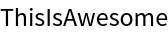In:=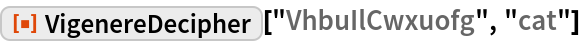Out=VigenereDecipher is related to VigenereCipher:

 In:=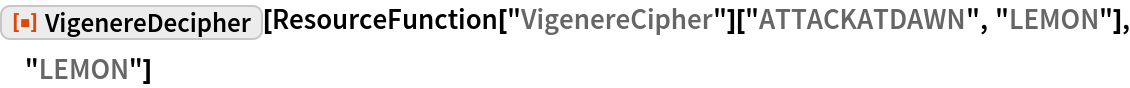Out=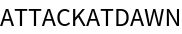Possible Issues

Spaces and the letters a and A in the key will not alter the enciphered message:

 In:=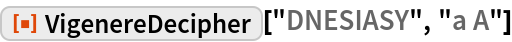Out=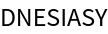The key should have valid letters and valid length:

 In:=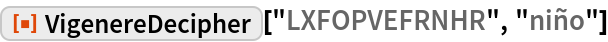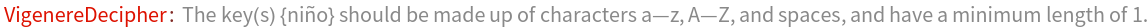Out=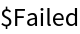In:=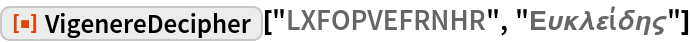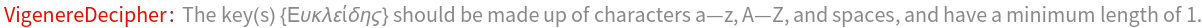Out=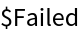In:=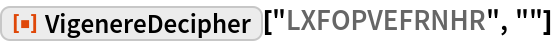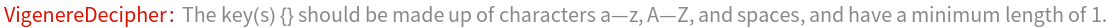Out=Two keys can "negate" each other:

 In:=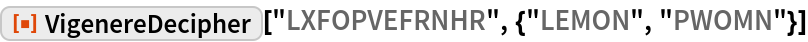Out=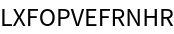Enciphering the keys with each other gives a string with only As:

 In:=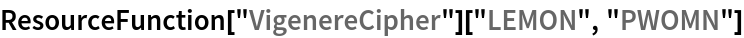Out=